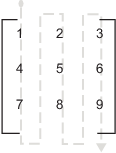Main Content

## Data Handling with TLC

### Matrix Parameters

MATLAB®, Simulink®, and the code generator use column-major ordering for array storage (1-D, 2-D, ...). The next element of an array in memory is accessed by incrementing the first index of the array. For example, these element pairs are stored sequentially in memory: `A(i)` and `A(i+1)`, `B(i,j)` and `B(i+1,j)`, `C(i,j,k)` and `C(i+1,j,k)`. For more information on the internal representation of MATLAB data, see MATLAB Data.

### Code Generator Matrix Parameters

Simulink and code generator internal data storage formatting differs from MATLAB internal data storage formatting only in the storage of complex number arrays. In MATLAB, the real and imaginary parts are stored in separate arrays. In the Simulink and code generator products they are stored in an "interleaved" format, where the numbers in memory alternate real, imaginary, real, imaginary, and so forth. This convention allows efficient implementations of small signals on Simulink lines and for Mux blocks and other "virtual" signal manipulation blocks (that is, they do not actively copy their inputs, merely the references to them).

The compiled model file, `model.rtw`, represents matrices as strings in MATLAB syntax, with no implied storage format. This is so you can copy the string out of an `.rtw` file and paste it into MATLAB code and have it recognized by MATLAB.

TLC declares Simulink block matrix parameters as scalar or 1-D array variables

```real_T scalar; real_T mat[ nRows * nCols ];```

where `real_T` can be an arbitrary data type supported by Simulink, and match the variable type given in the model file.

For example, the 3-by-3 matrix in the Look-Up Table (2-D) block

```1 2 3 4 5 6 7 8 9```

is stored in `model.rtw` as

```Parameter { Name "OutputValues" Value Matrix(3,3) [[1.0, 2.0, 3.0]; [4.0, 5.0, 6.0]; [7.0, 8.0, 9.0];] String "t" StringType "Variable" ASTNode { IsNonTerminal 0 Op SL_NOT_INLINED ModelParameterIdx 3 } }```

and results in this definition in `model.h`

```typedef struct Parameters_tag { real_T s1_Look_Up_Table_2_D_Table; /* Variable:s1_Look_Up_Table_2_D_Table * External Mode Tunable:yes * Referenced by block: * <S1>/Look-Up Table (2-D */ [ ... other parameter definitions ... ] } Parameters;```

The `model.h` file declares the actual storage for the matrix parameter and you can see that the format is column-major. That is, read down the columns, then across the rows.```Parameters model_P = { /* 3 x 3 matrix s1_Look_Up_Table_2_D_Table */ { 1.0, 4.0, 7.0, 2.0, 5.0, 8.0, 3.0, 6.0, 9.0 }, [ ... other parameter declarations ...] };```

TLC accesses matrix parameters via `LibBlockMatrixParameter` and `LibBlockMatrixParameterAddr`, where

```LibBlockMatrixParameter(OutputValues, "", "", 0, "", "", 1)``` returns `"model_P.s1_Look_Up_Table_2_D_Table[nRows]"` (automatically optimized from `"[0+nRows*1]"`) and

```LibBlockMatrixParameterAddr(OutputValues, "", "", 0, "", "", 1)``` returns `"&model_P.s1_Look_Up_Table_2_D_Table[nRows]"` for both inlined and noninlined block TLC code.

Matrix parameters are like other TLC parameters. Only those parameters explicitly accessed by a TLC library function during code generation are placed in the parameters structure. So, following the example, `s1_Look_Up_Table_2_D_Table` is not declared unless `LibBlockParameter` or `LibBlockParameterAddr` explicitly access it.

Download guide